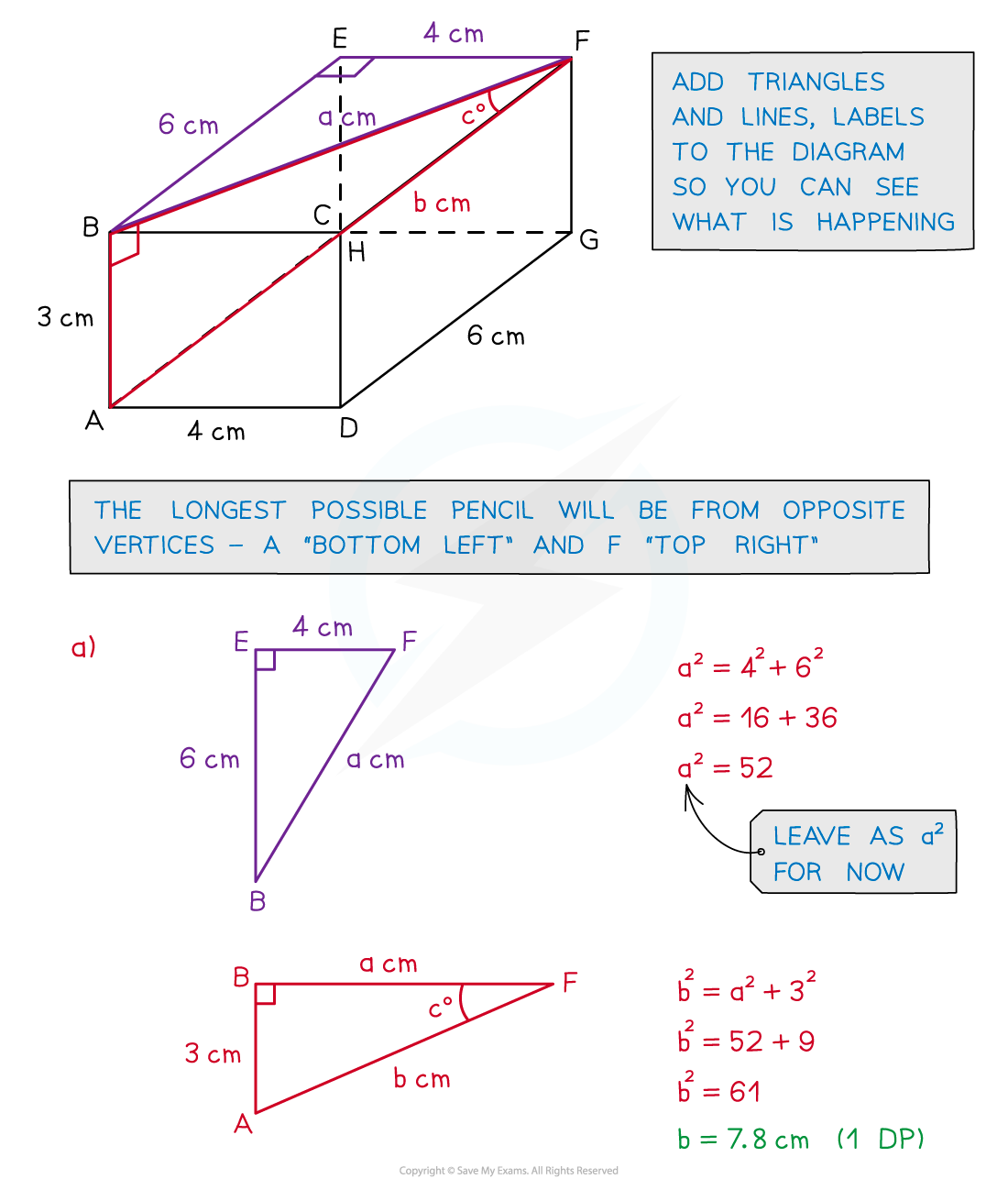# Edexcel IGCSE Maths 复习笔记 4.9.2 3D Pythagoras & SOHCAHTOA

Edexcel IGCSE Maths 复习笔记 4.9.2 3D Pythagoras & SOHCAHTOA

#### What are Pythagoras & SOHCAHTOA?

• Pythagoras’ Theorem helps us find missing side lengths of a right-angled triangle
• It is also frequently used for finding the distance (or length) of a line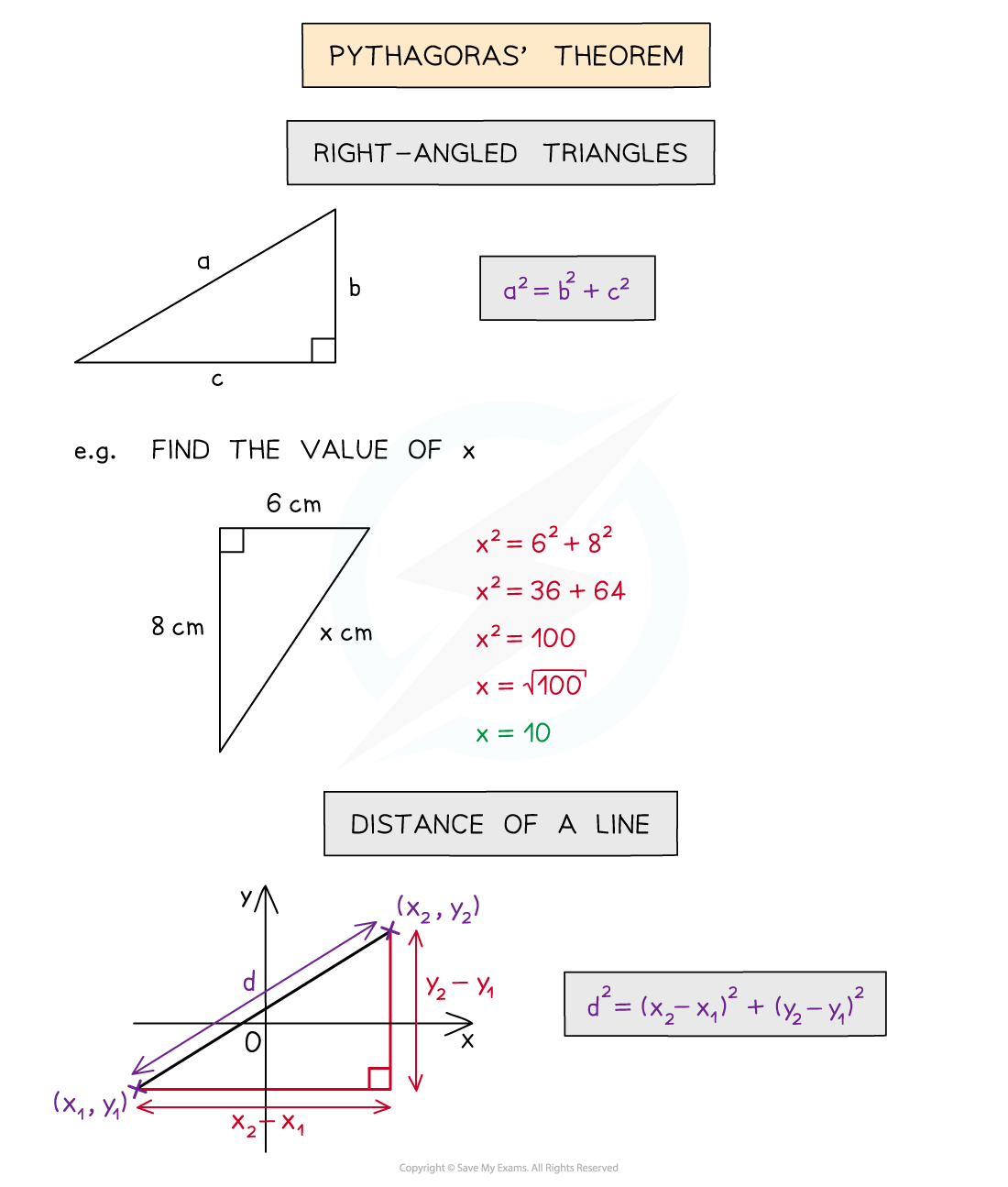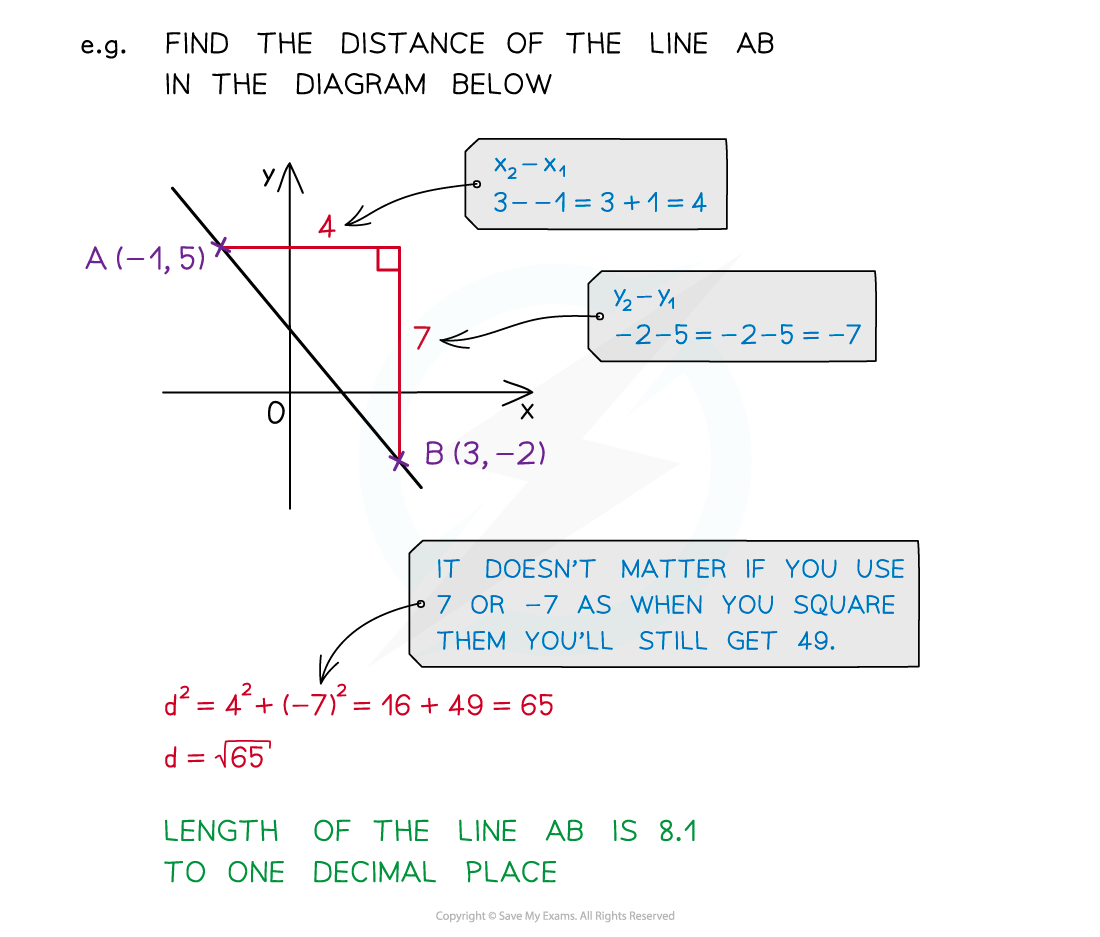• SOHCAHTOA is an acronym for the three trigonometric ratios that connect angles (θ) and sides (Opposite, Hypotenuse and Adjacent) in a right-angled triangle
• Sine – SOH – sin θ = O ÷ H
• Cosine – CAH – cos θ = A ÷ H
• Tangent – TOA – tan θ = O ÷ A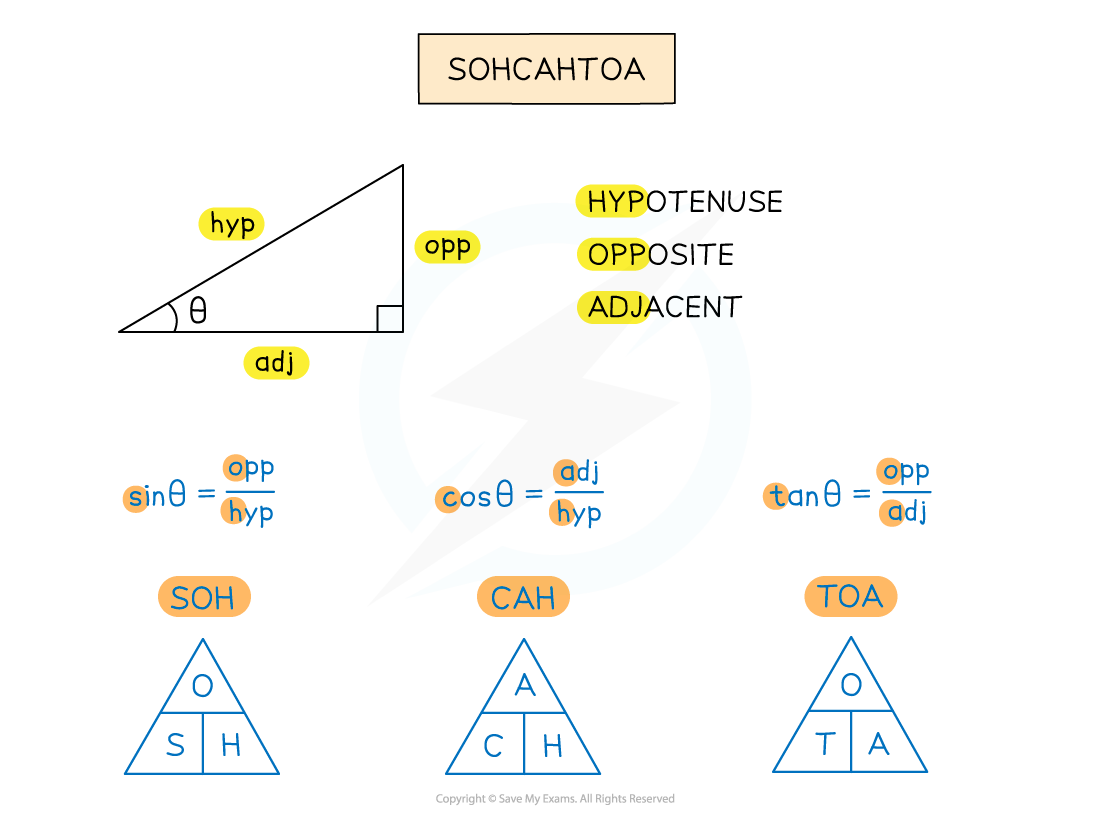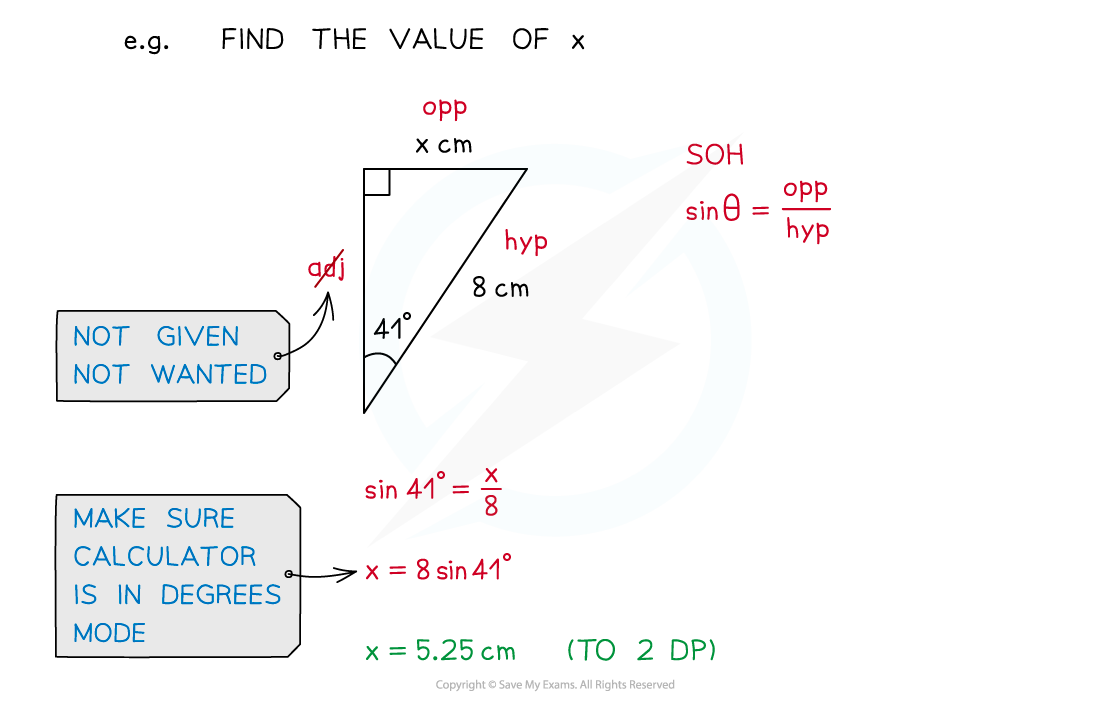#### How does Pythagoras work in 3D?

• 3D shapes can often be broken down into several 2D shapes
• For example nets and surface area

• With Pythagoras’ Theorem problems you will be specifically looking for right‑angled triangles
• The right-angled triangles you need will have two known sides and one unknown side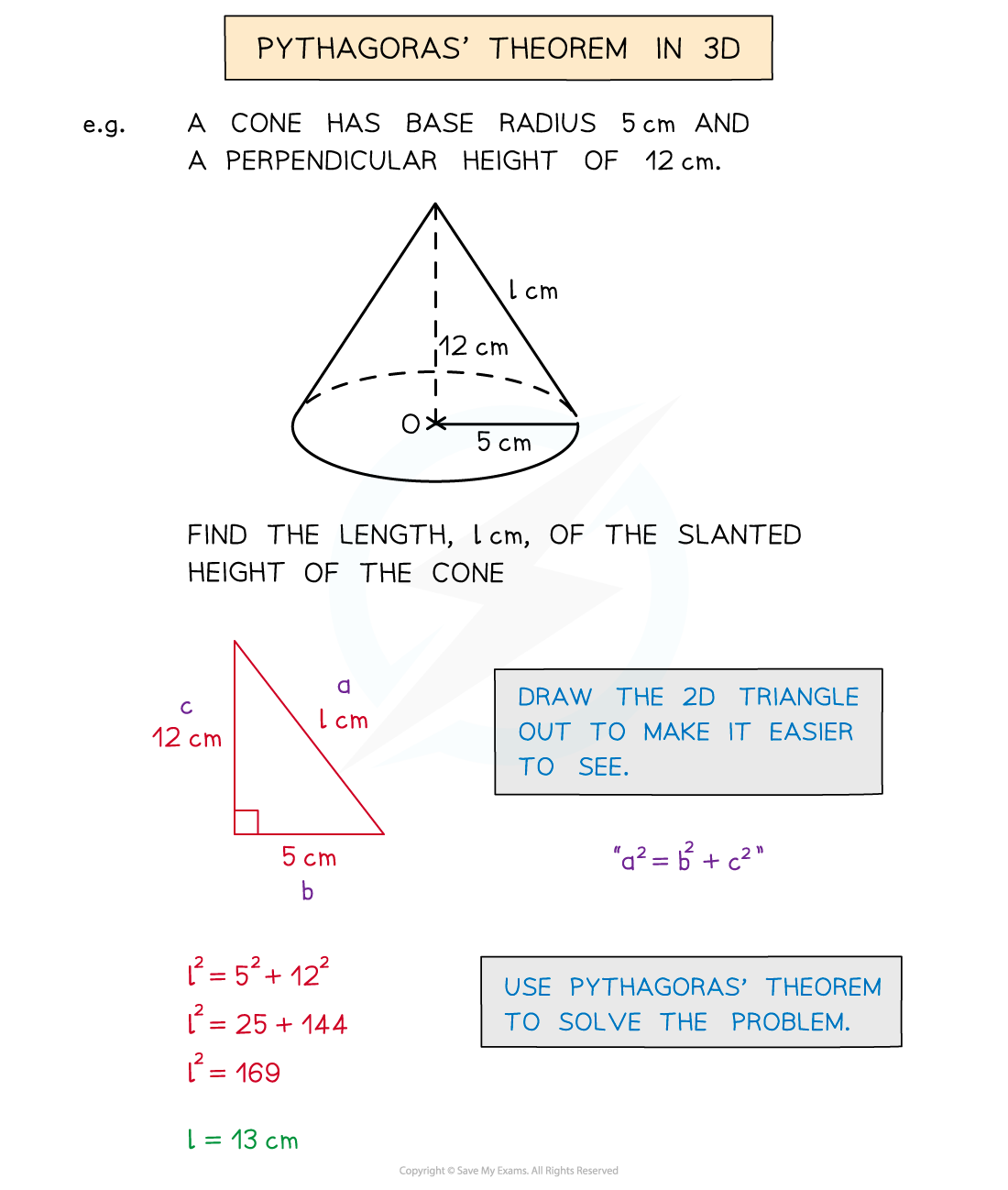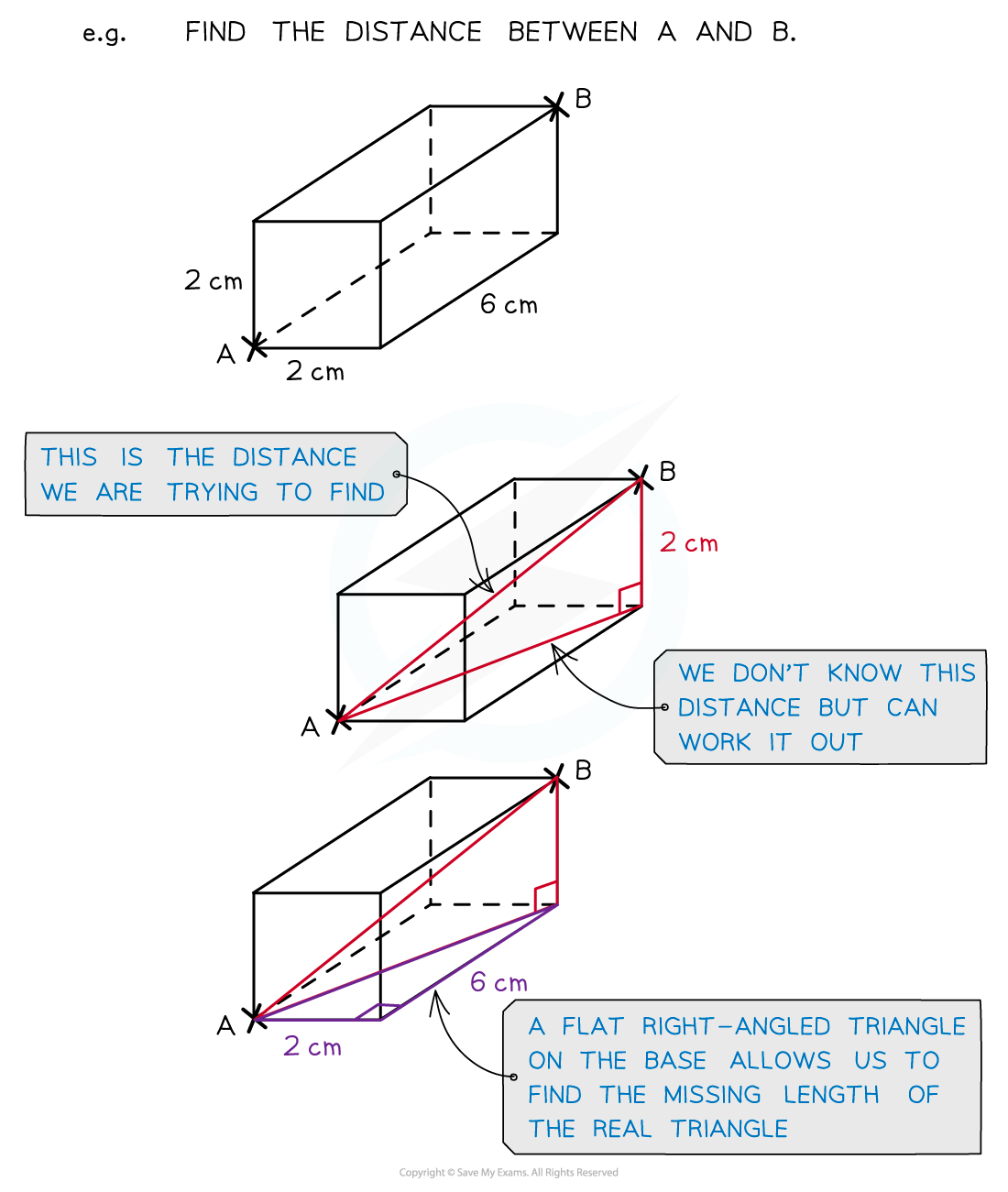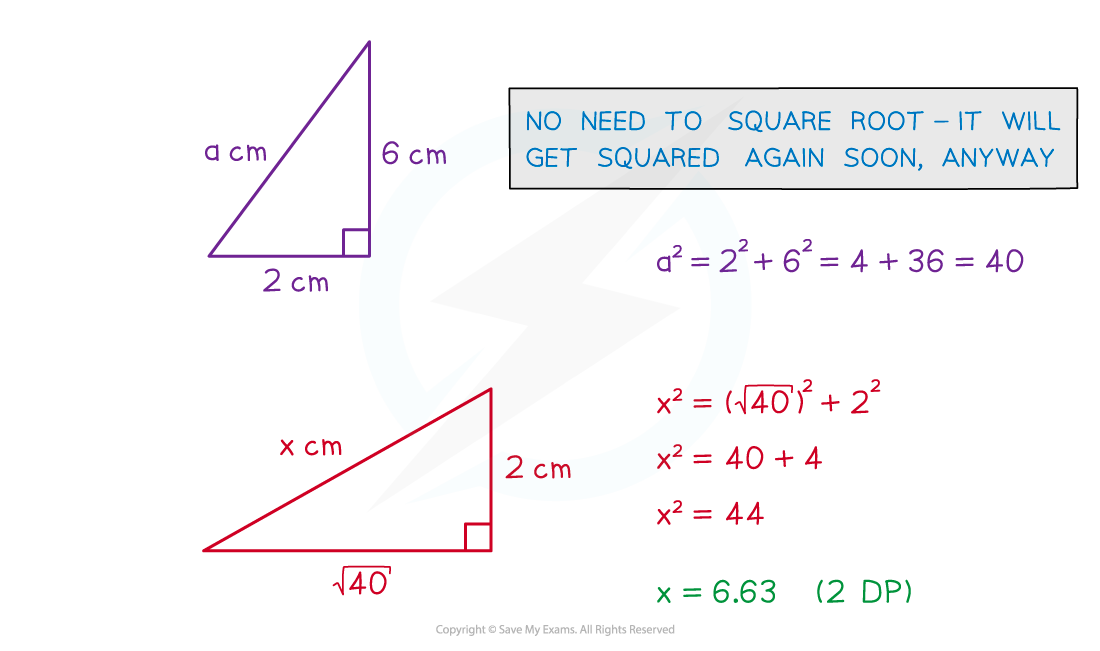• There is a 3D version of the Pythagoras’ Theorem formula
• d2 = x2 + y2 + z2

• However it is usually far easier to see a problem by splitting it into two or more 2D problems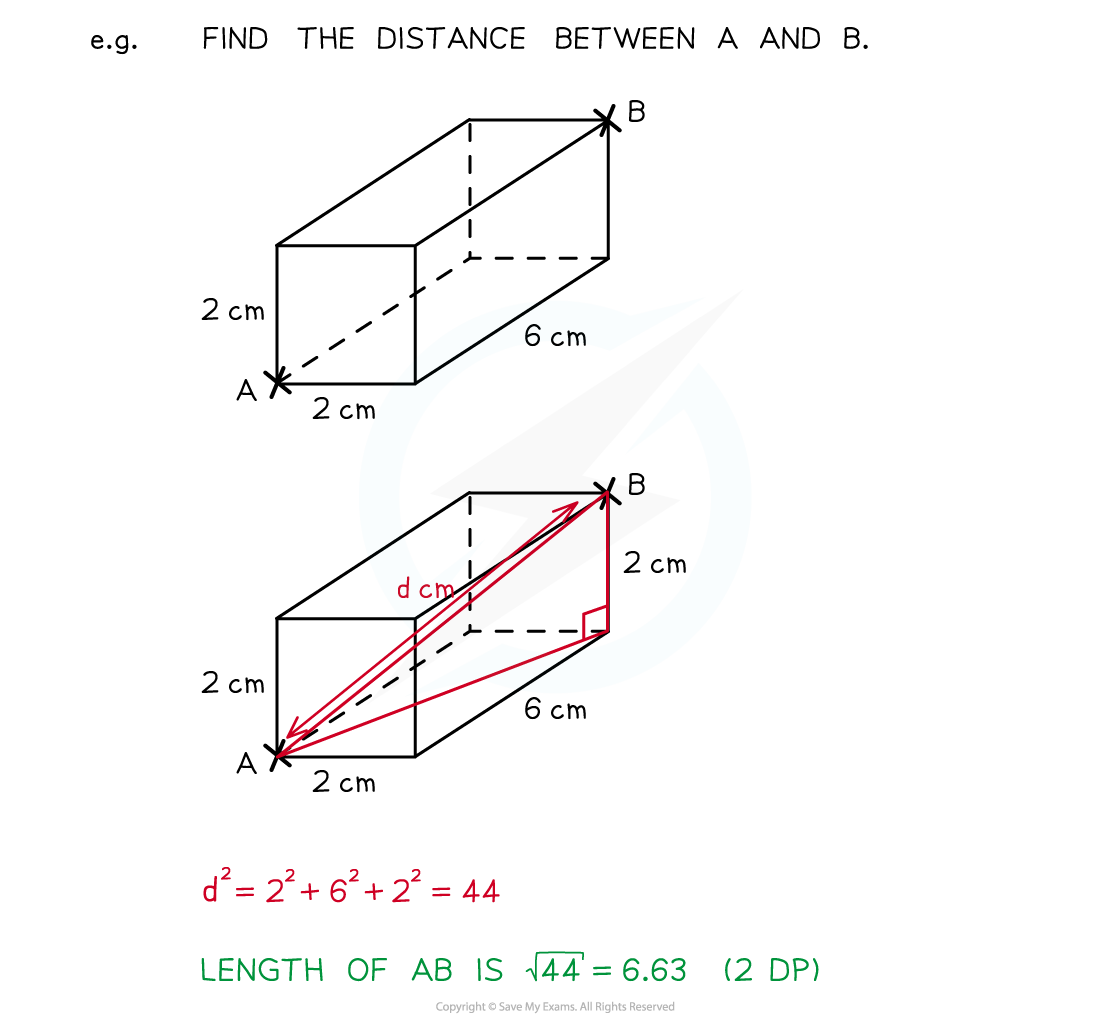#### How does SOHCAHTOA work in 3D?

• Again look for a combination of right-angled triangles that would lead to the missing angle or side
• The angle you are working with can be awkward in 3D
• The angle between a line and a plane is not obvious
• If unsure, put a point on the line and draw a new line to the planeThis should create a right-angled triangle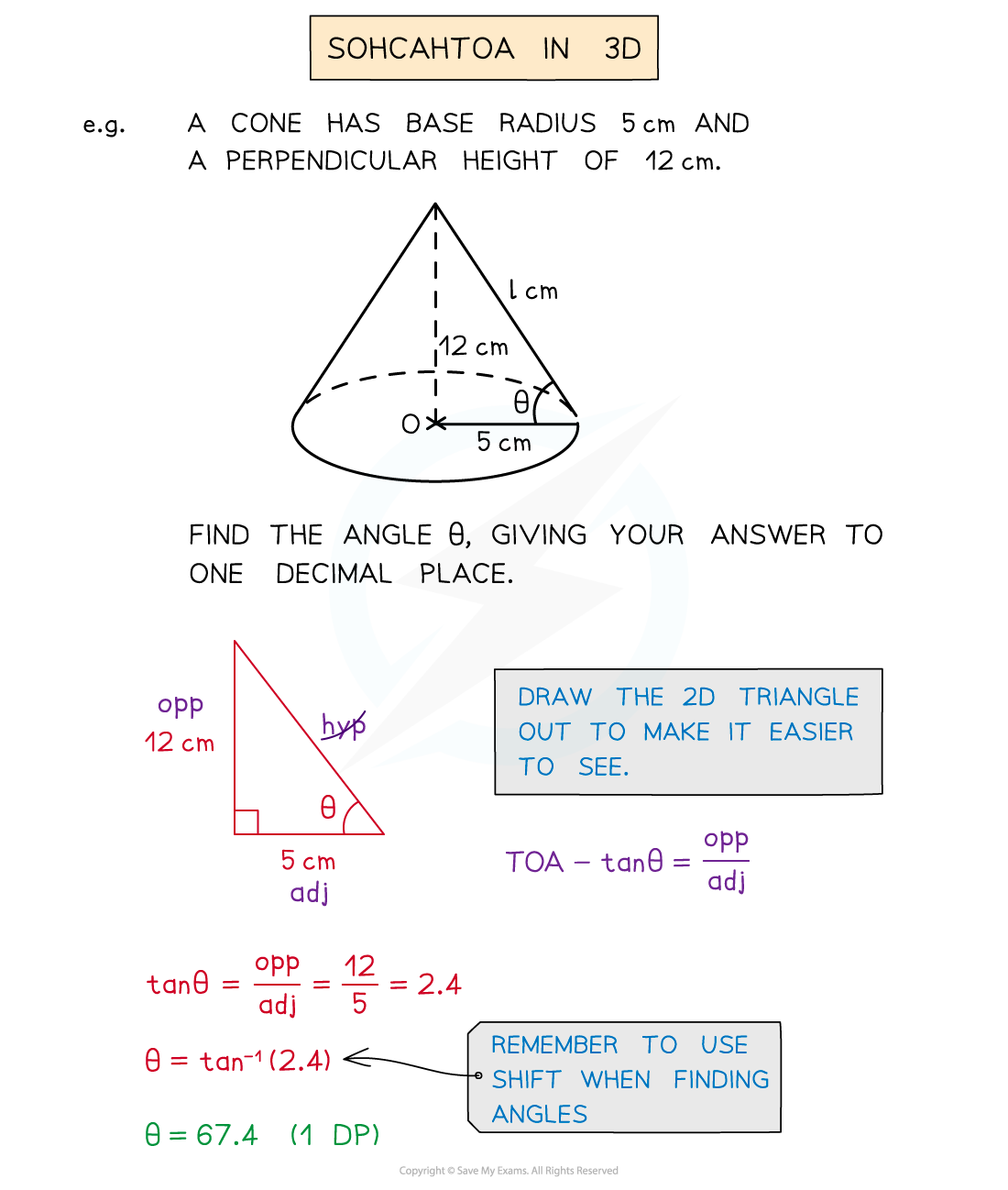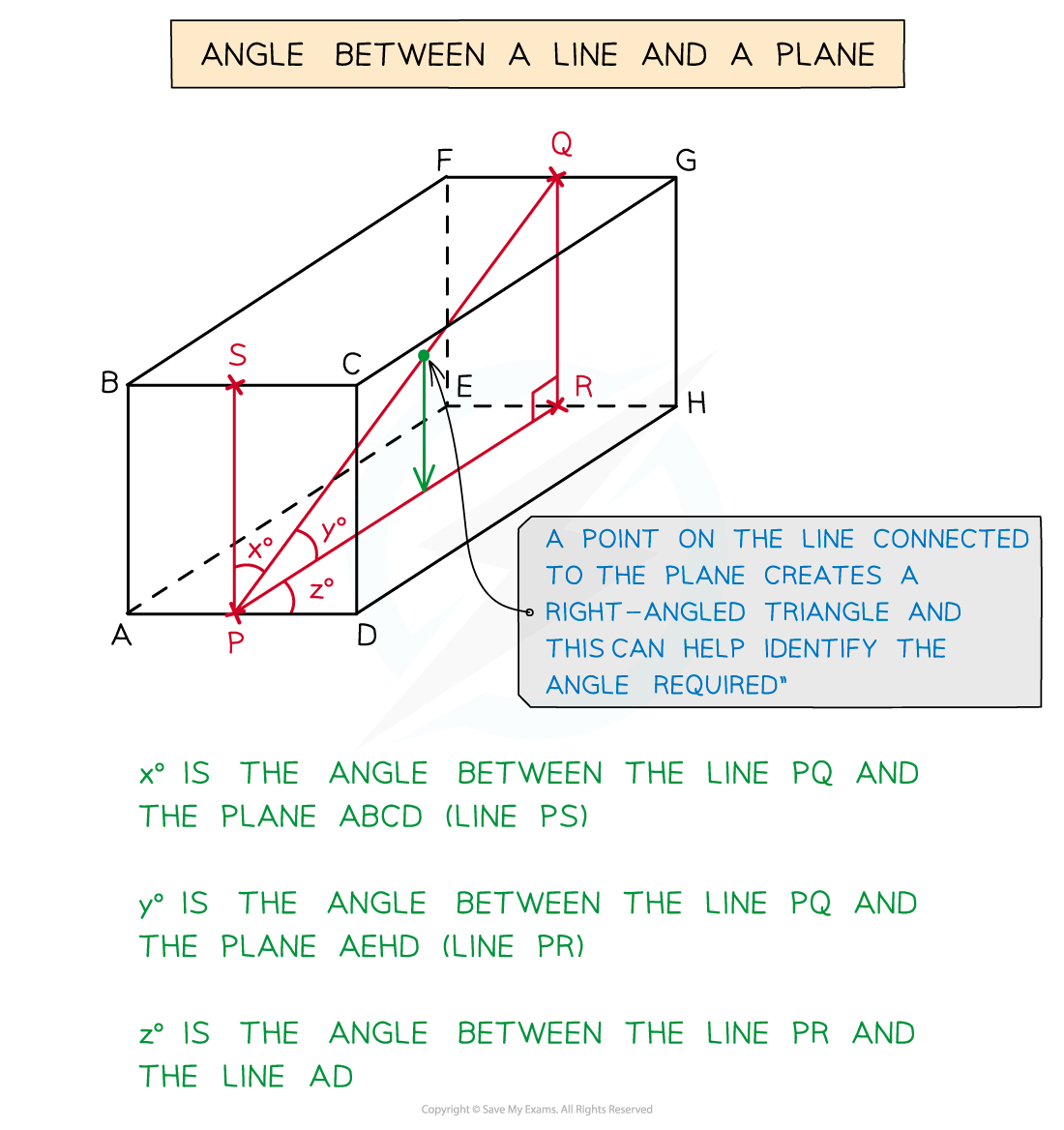• Once you have your 2D triangle(s) you can begin to solve problems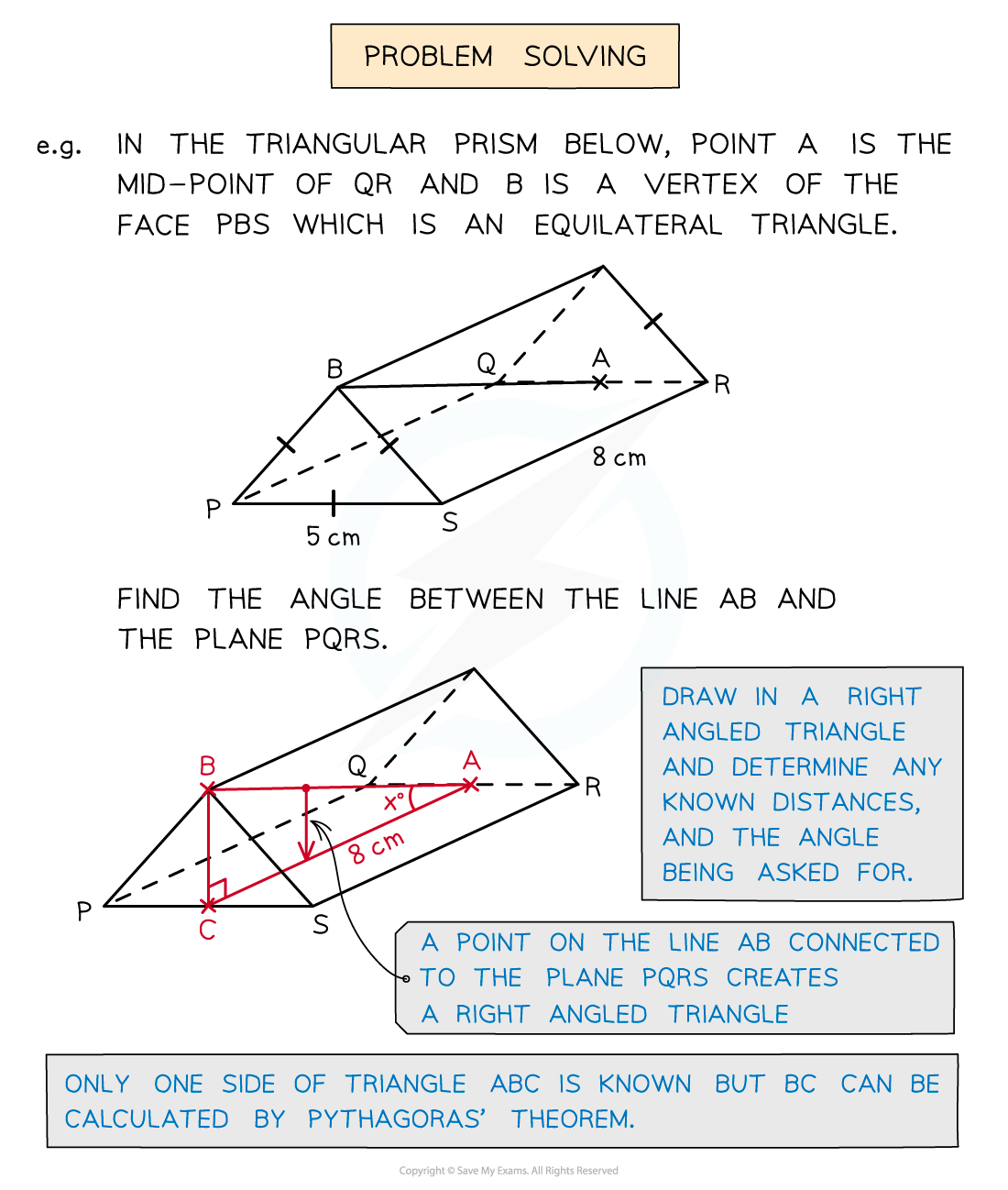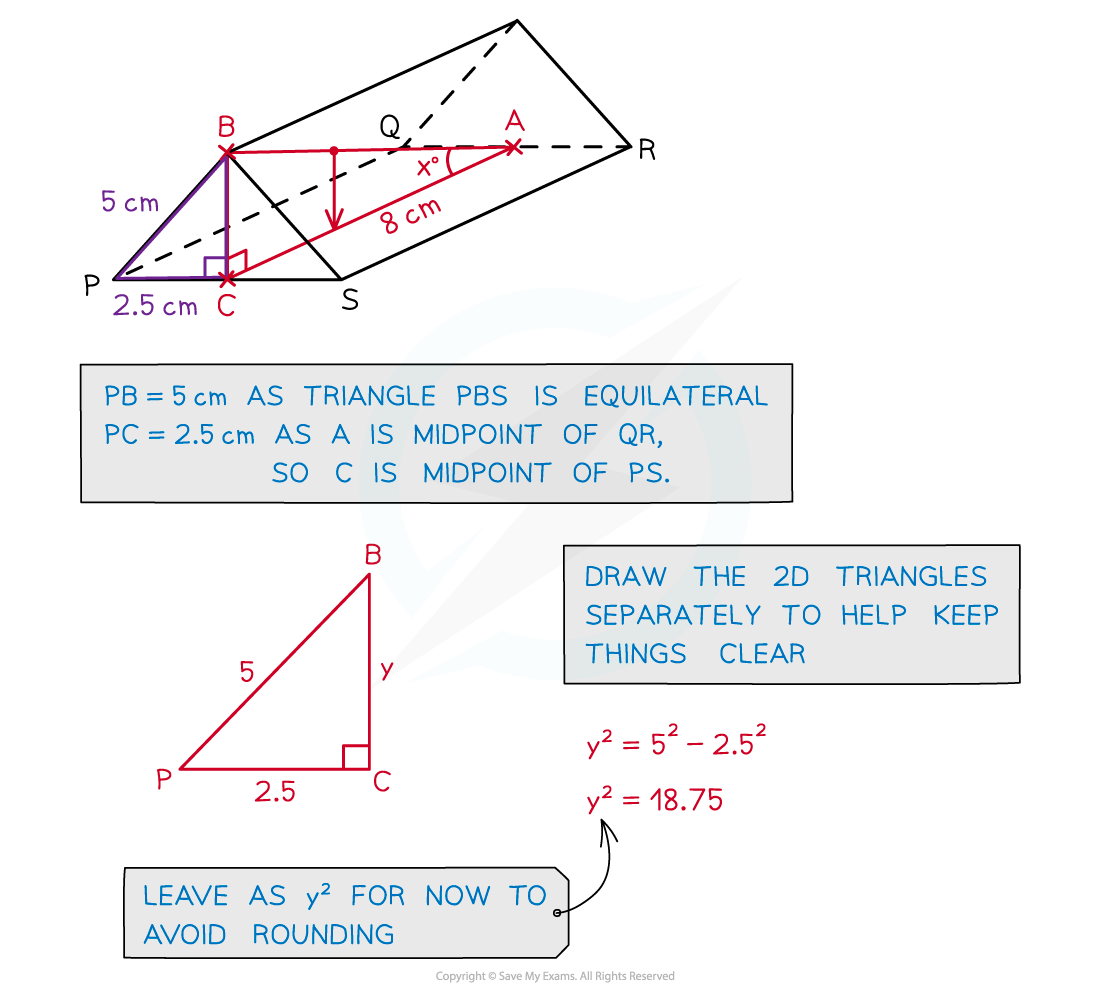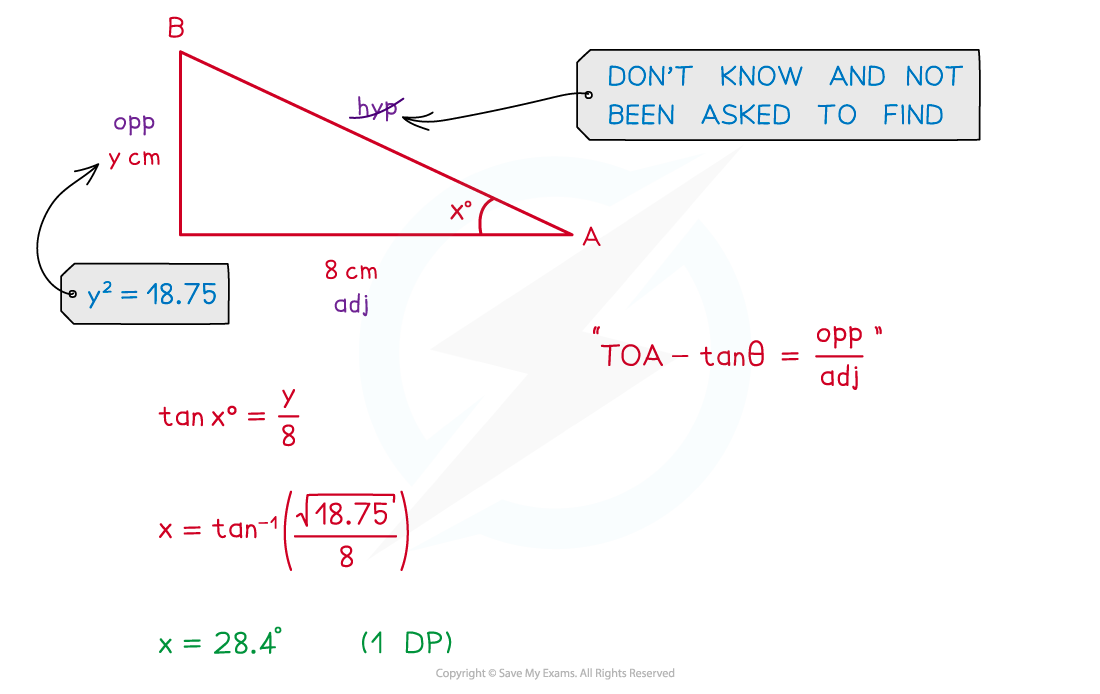#### Exam Tip

Add lines/triangles/etc. to any given diagram to help you see the problem and draw any 2D triangles separately as a 3D diagram can get hard to follow.

#### Worked Example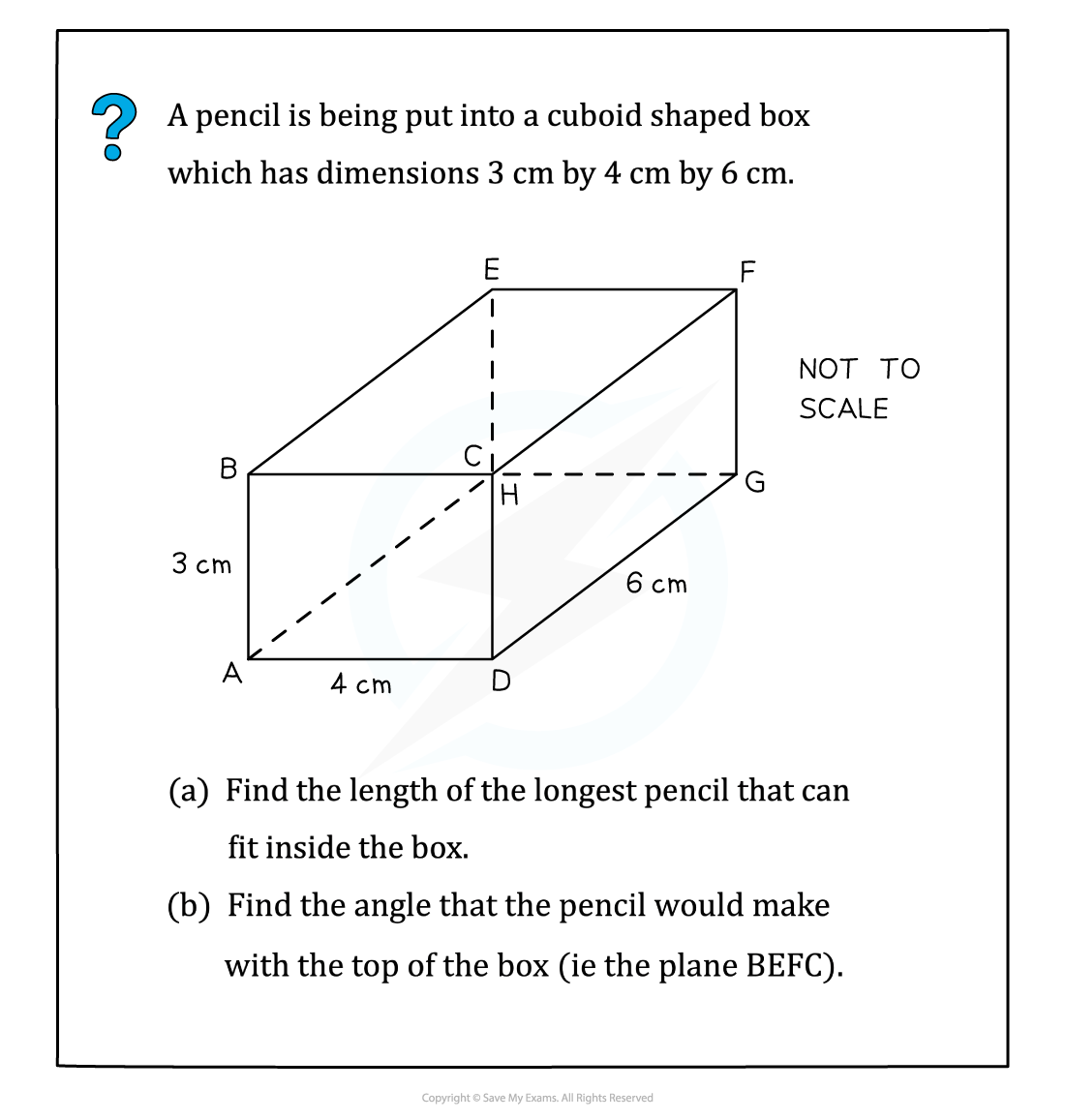#### Worked Example